# 基于ROS的机器人建图与导航仿真全过程新机器视觉 |22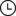2023-03-08 07:46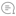0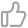00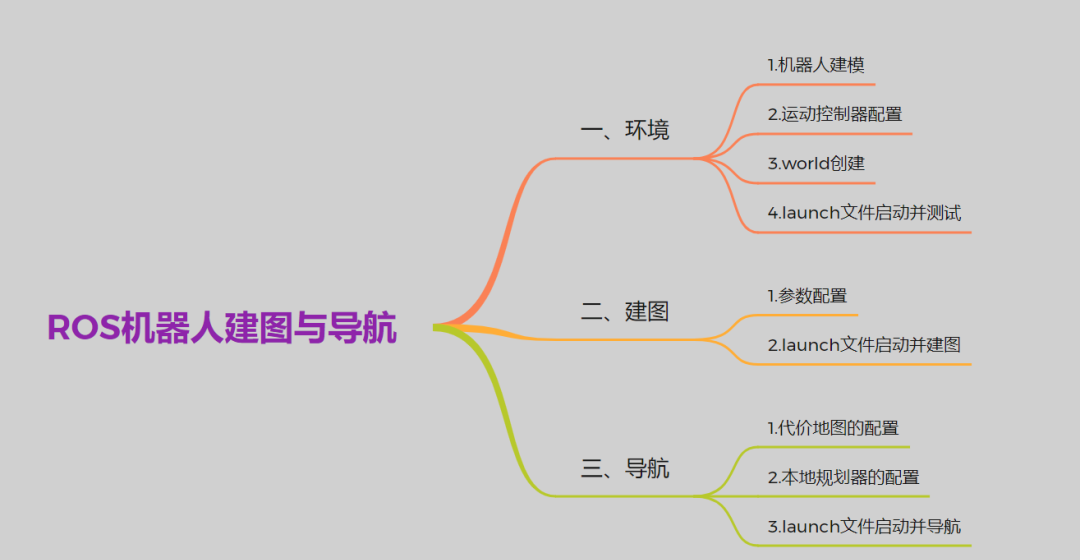1.机器人建模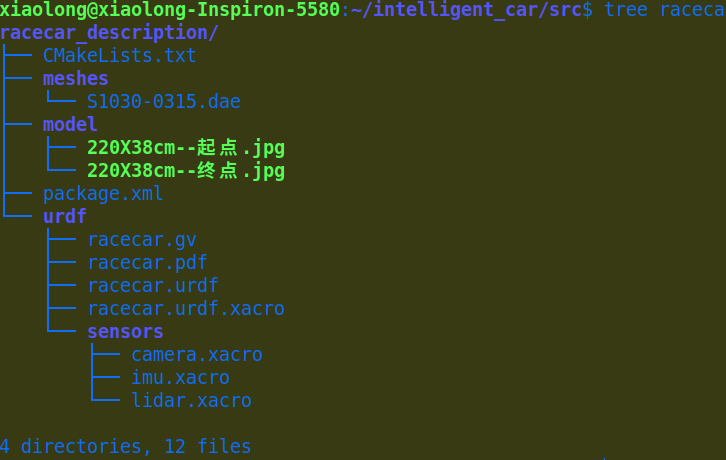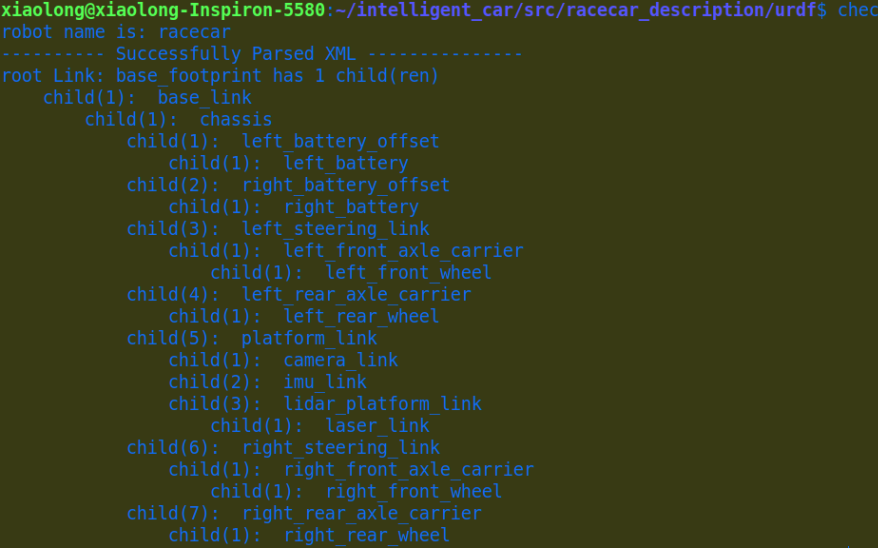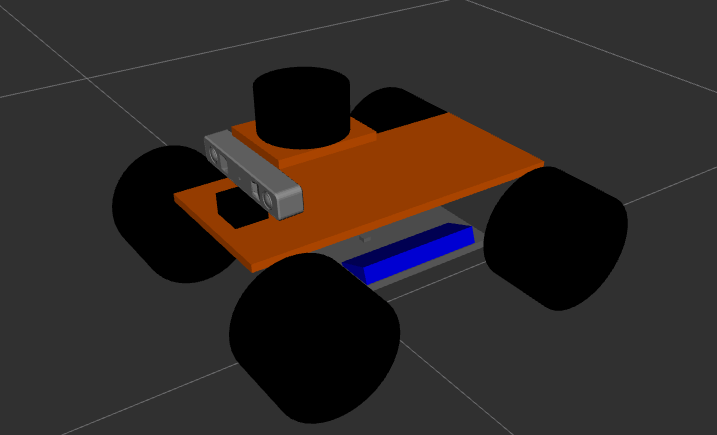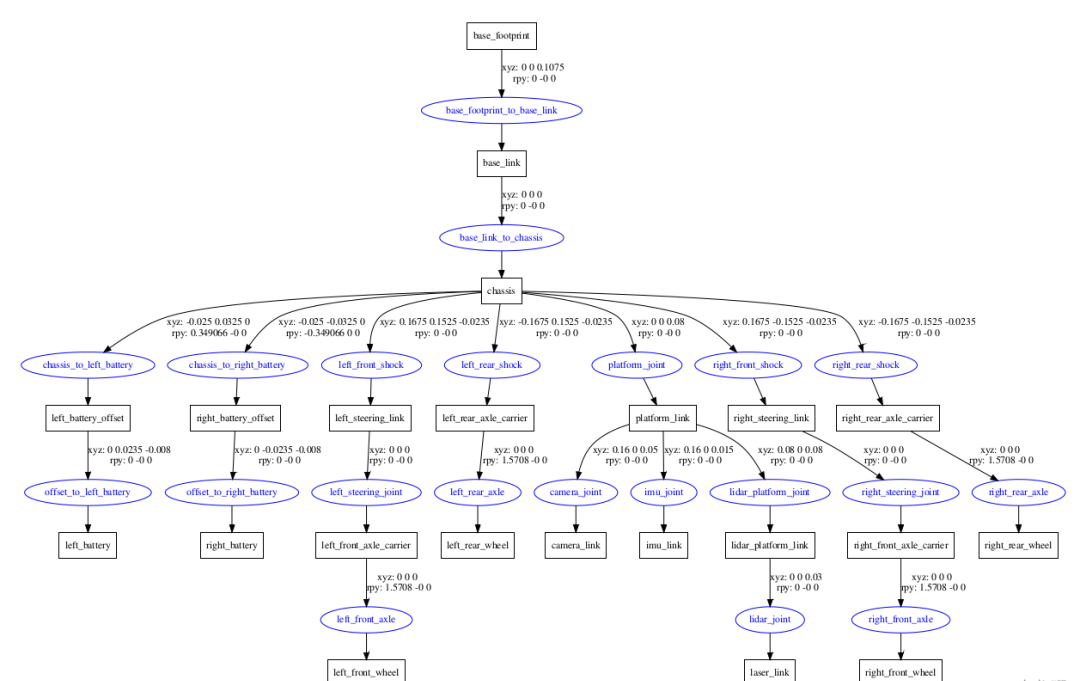2.运动控制器配置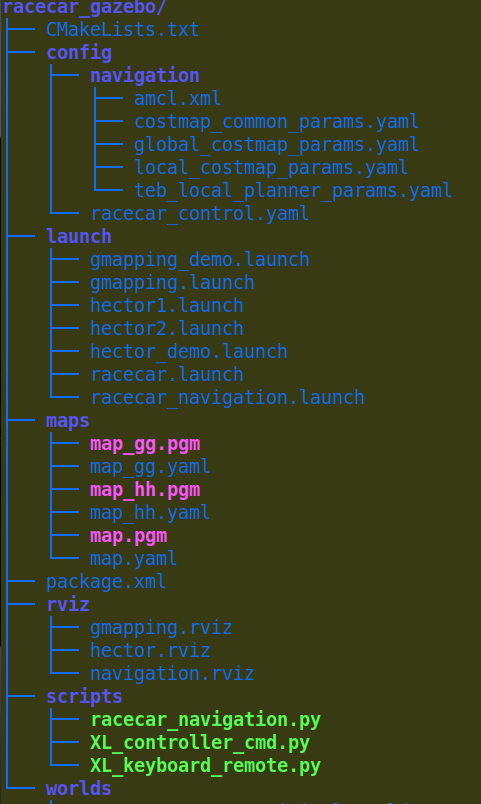``racecar:``  left_rear_wheel_velocity_controller:``    type: effort_controllers/JointVelocityController``    joint: left_rear_axle``    pid: {p: 1000.0, i: 0.00, d: 0.0}``  right_rear_wheel_velocity_controller:``    type: effort_controllers/JointVelocityController``    joint: right_rear_axle ``    pid: {p: 1000.0, i: 0.00, d: 0.0}``  left_front_wheel_velocity_controller:``    type: effort_controllers/JointVelocityController``    joint: left_front_axle``    pid: {p: 1000.0, i: 0.00, d: 0.0}``  right_front_wheel_velocity_controller:``    type: effort_controllers/JointVelocityController``    joint: right_front_axle``    pid: {p: 1000.0, i: 0.00, d: 0.0}``    ``  left_steering_hinge_position_controller:``    type: effort_controllers/JointPositionController``    joint: left_steering_joint``    pid: {p: 10000.0, i: 0.1, d: 500.0}``  ``  right_steering_hinge_position_controller:``    type: effort_controllers/JointPositionController``    joint: right_steering_joint``    pid: {p: 10000.0, i: 0.1, d: 500.0}``  joint_state_controller:``    type: joint_state_controller/JointStateController``    publish_rate: 50``3.world创建

world的创建方法有很多了，你可以自己画一个世界，也可以导入，这里可以给大家安利另外一个仿真神器：Webots，你会发现不一样的东西。4.launch文件启动并测试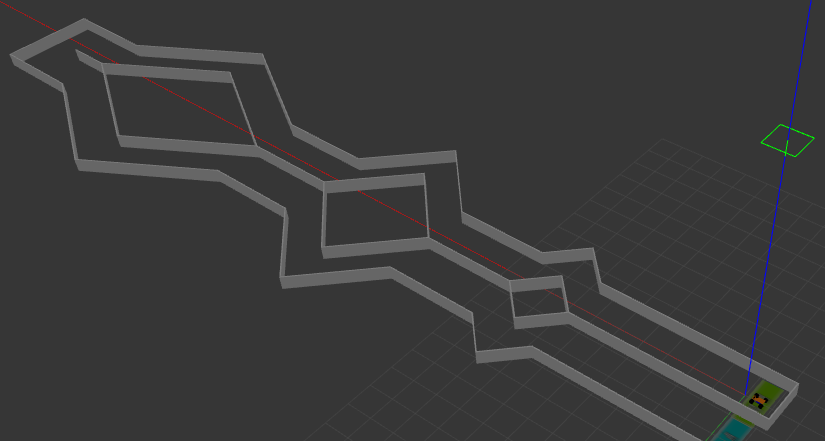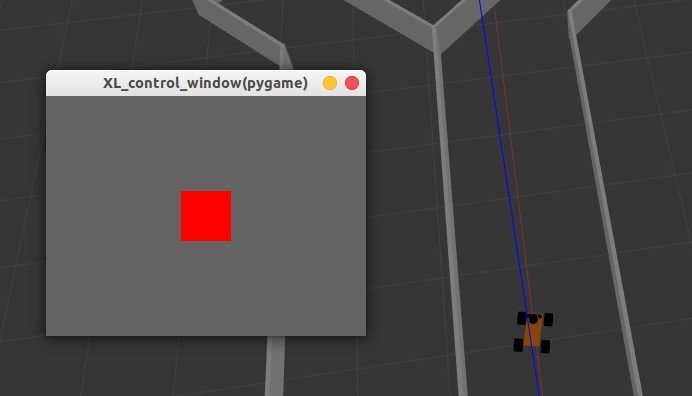1.参数配置

``<launch>``    <arg name="scan_topic" default="scan" />``    <node pkg="gmapping" type="slam_gmapping" name="slam_gmapping" output="screen" clear_params="true">``        <param name="odom_frame" value="odom"/>``        <param name="map_update_interval" value="5.0"/>``        <!-- Set maxUrange < actual maximum range of the Laser -->``        <param name="maxRange" value="5.0"/>``        <param name="maxUrange" value="4.5"/>``        <param name="sigma" value="0.05"/>``        <param name="kernelSize" value="1"/>``        <param name="lstep" value="0.05"/>``        <param name="astep" value="0.05"/>``        <param name="iterations" value="5"/>``        <param name="lsigma" value="0.075"/>``        <param name="ogain" value="3.0"/>``        <param name="lskip" value="0"/>``        <param name="srr" value="0.01"/>``        <param name="srt" value="0.02"/>``        <param name="str" value="0.01"/>``        <param name="stt" value="0.02"/>``        <param name="linearUpdate" value="0.5"/>``        <param name="angularUpdate" value="0.436"/>``        <param name="temporalUpdate" value="-1.0"/>``        <param name="resampleThreshold" value="0.5"/>``        <param name="particles" value="80"/>``        <param name="xmin" value="-1.0"/>``        <param name="ymin" value="-1.0"/>``        <param name="xmax" value="1.0"/>``        <param name="ymax" value="1.0"/>``        <param name="delta" value="0.05"/>``        <param name="llsamplerange" value="0.01"/>``        <param name="llsamplestep" value="0.01"/>``        <param name="lasamplerange" value="0.005"/>``        <param name="lasamplestep" value="0.005"/>``        <remap from="scan" to="\$(arg scan_topic)"/>``    </node>``</launch>``

``<launch>``    <include file="\$(find racecar_gazebo)/launch/gmapping.launch"/>``    <include file="\$(find racecar_gazebo)/launch/racecar.launch"/>``  ``    <!-- keyboard_remote node -->``    <node name="XL_keyboard_remote" pkg="racecar_gazebo" type="XL_keyboard_remote.py" output="screen">``    </node>``    <!-- 启动rviz -->``    <node pkg="rviz" type="rviz" name="rviz" args="-d \$(find racecar_gazebo)/rviz/gmapping.rviz"/>``</launch>``

2.launch文件启动并建图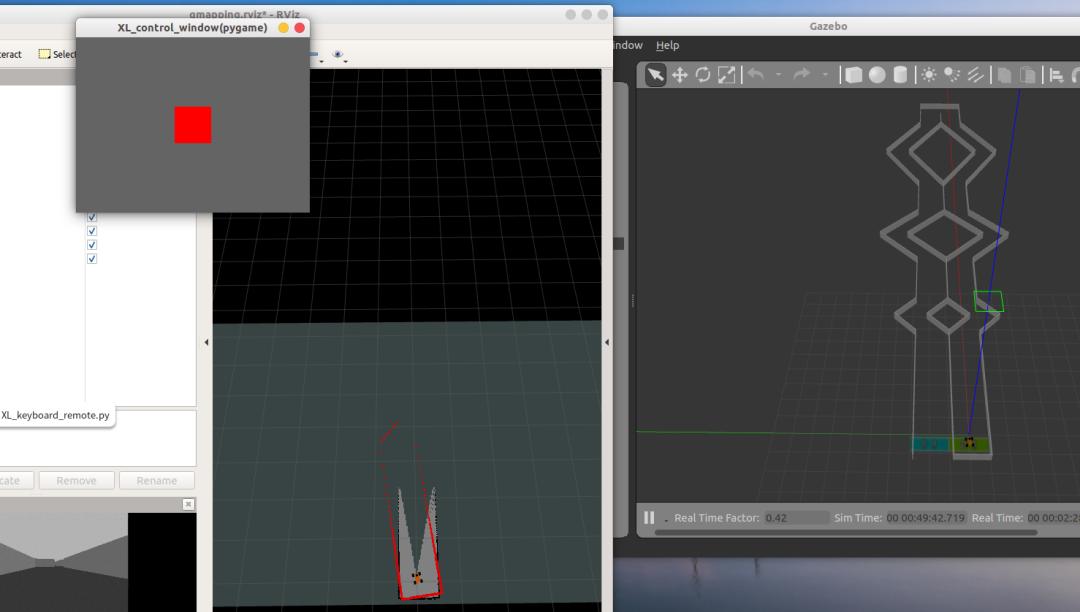最后建成的地图我们需要及时保存。保存的地图一共有两个文件，map.pgm和map.yaml。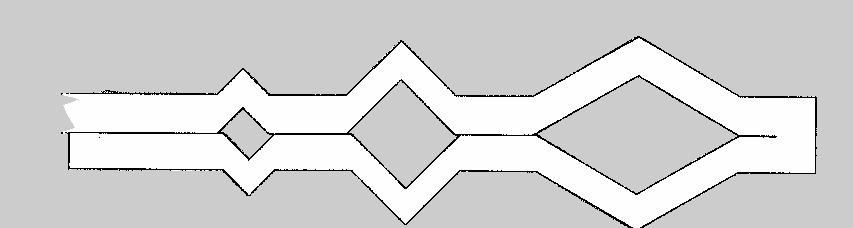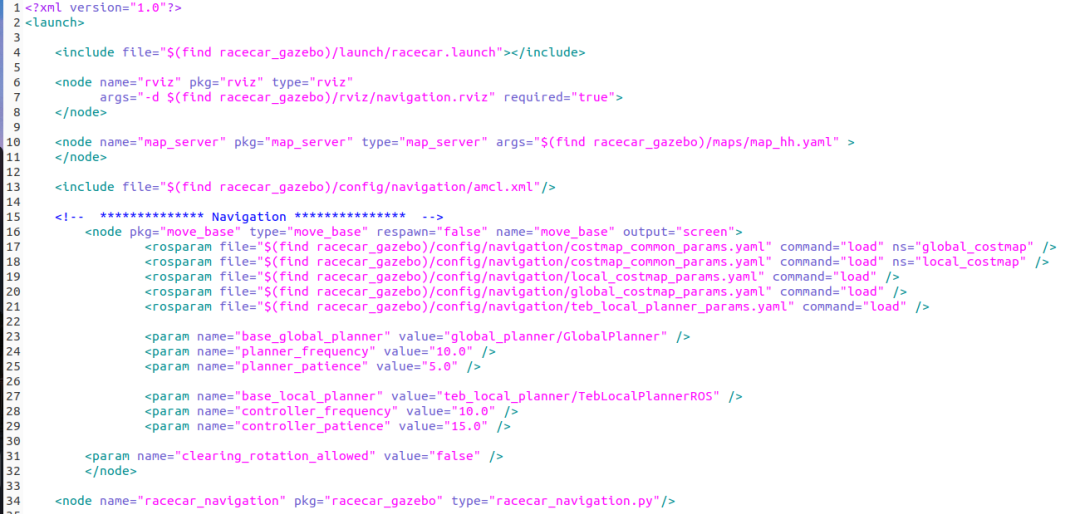1.代价地图的配置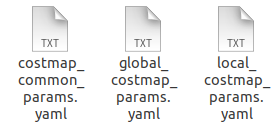2.本地规划器的配置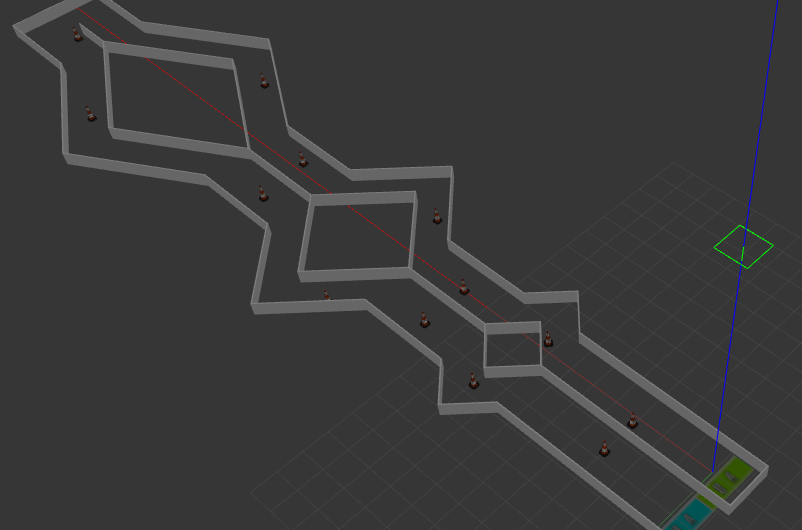3.launch文件启动并导航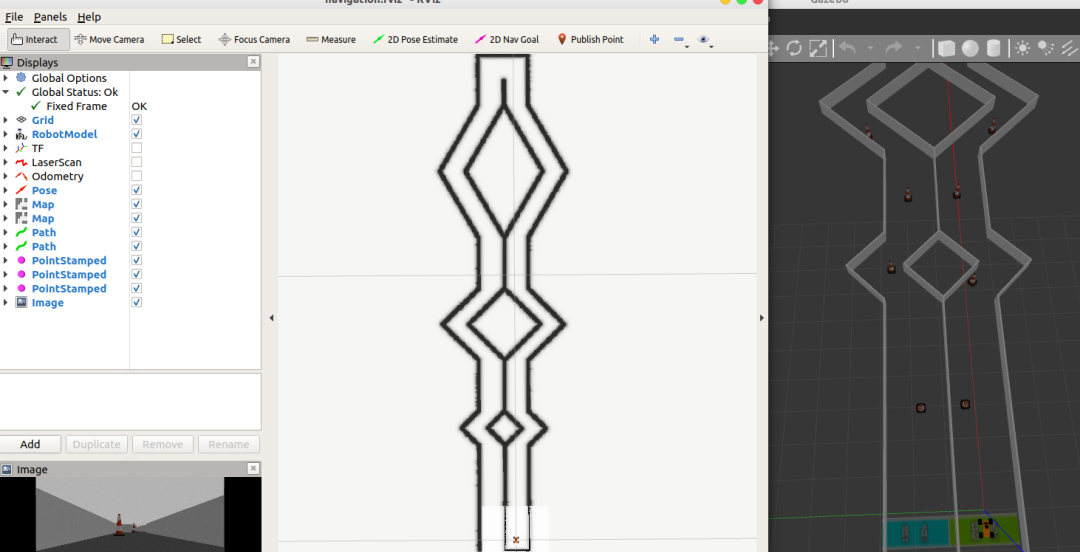通过rviz上2D Nav Goal来给机器人发布导航终点信息，可以看出机器人已经开始实时扫描信息并规划路径向着终点出发啦：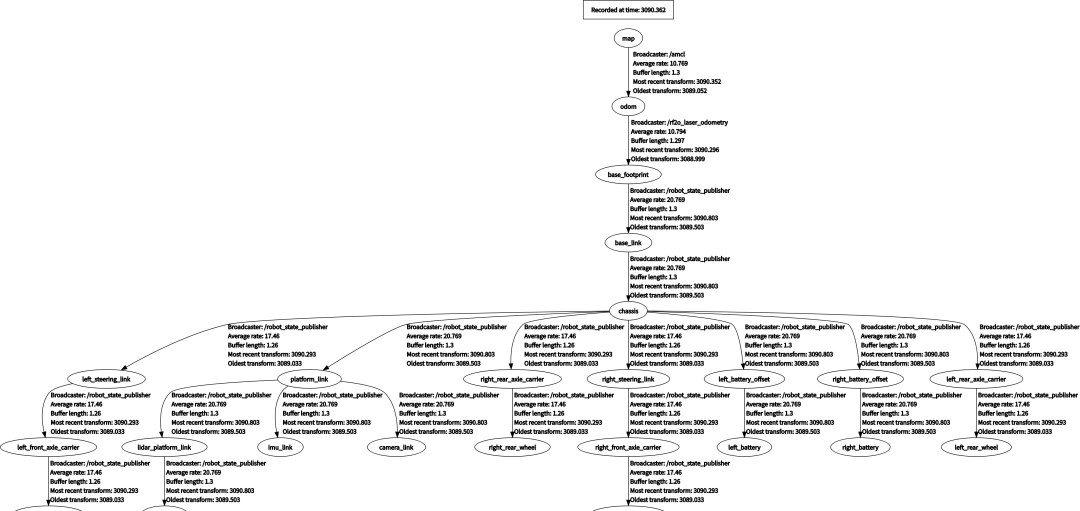也可以通过rosrun rqt_graph rqt_graph来查看导航过程中的各节点：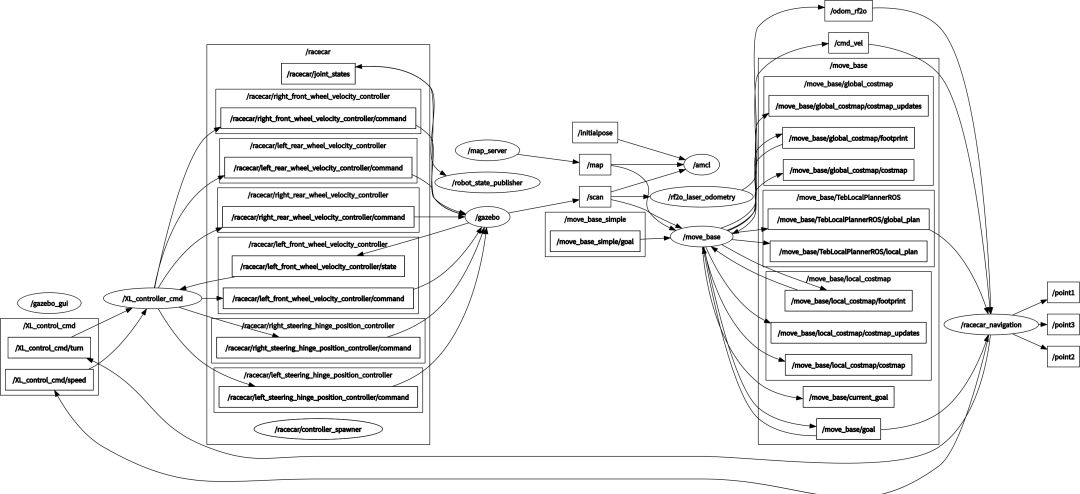—THE END—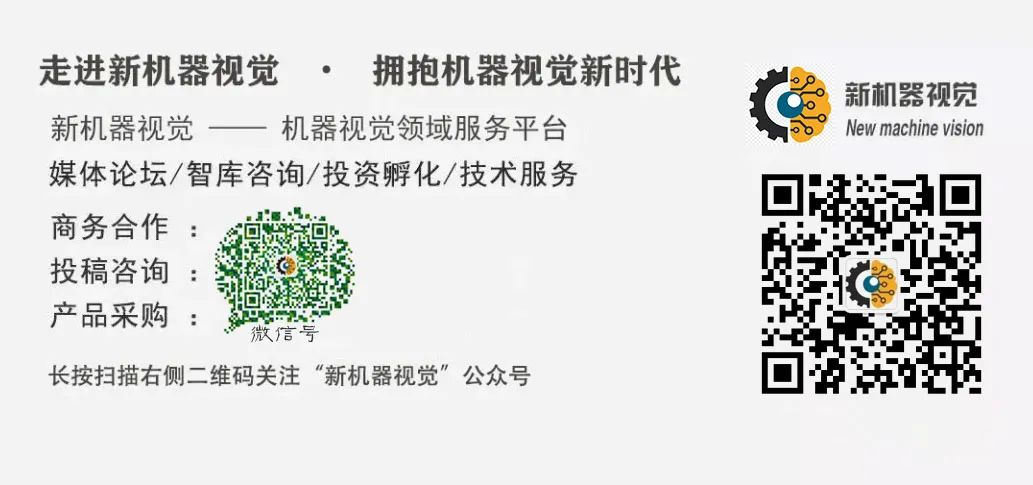00

暂无评论~~
Ctrl+Enter